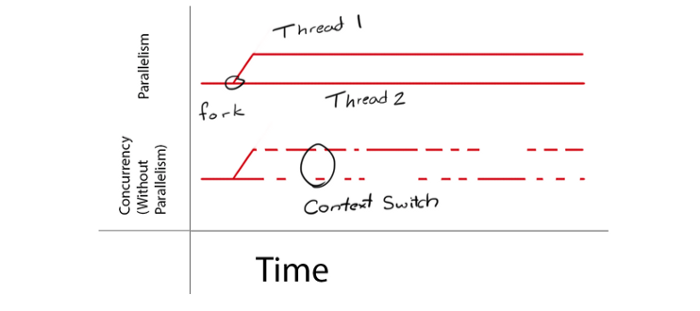## 数据竞争

``````var balance int

func Deposit(amount int) {
balance = balance + amount
}
func Balance() int {
return balance
}
``````

``````// Alice:
go func() {
bank.Deposit(200)                // A1
fmt.Println("=", bank.Balance()) // A2
}()

// Bob:
go bank.Deposit(100)                 // B
``````

## sync.Mutex 互斥锁

``````var (
sema    = make(chan struct{}, 1) // a binary semaphore guarding balance
balance int
)

func Deposit(amount int) {
sema <- struct{}{} // acquire token
balance = balance + amount
<-sema // release token
}

func Balance() int {
sema <- struct{}{} // acquire token
b := balance
<-sema // release token
return b
}
``````

``````import "sync"

var (
mu      sync.Mutex // guards balance
balance int
)

func Deposit(amount int) {
mu.Lock()
defer mu.Unlock()
balance = balance + amount
}

func Balance() int {
mu.Lock()
defer mu.Unlock()
b := balance
return b
}
``````

## sync.RWMutex 读写锁

``````import "sync"

var (
mu      sync.RWMutex
balance int
)

func Deposit(amount int) {
mu.Lock()
defer mu.Unlock()
balance = balance + amount
}

func Balance() int {
mu.RLocker()
defer mu.RUnlock()
b := balance
return b
}
``````

## 参考

9.2. sync.Mutex互斥锁